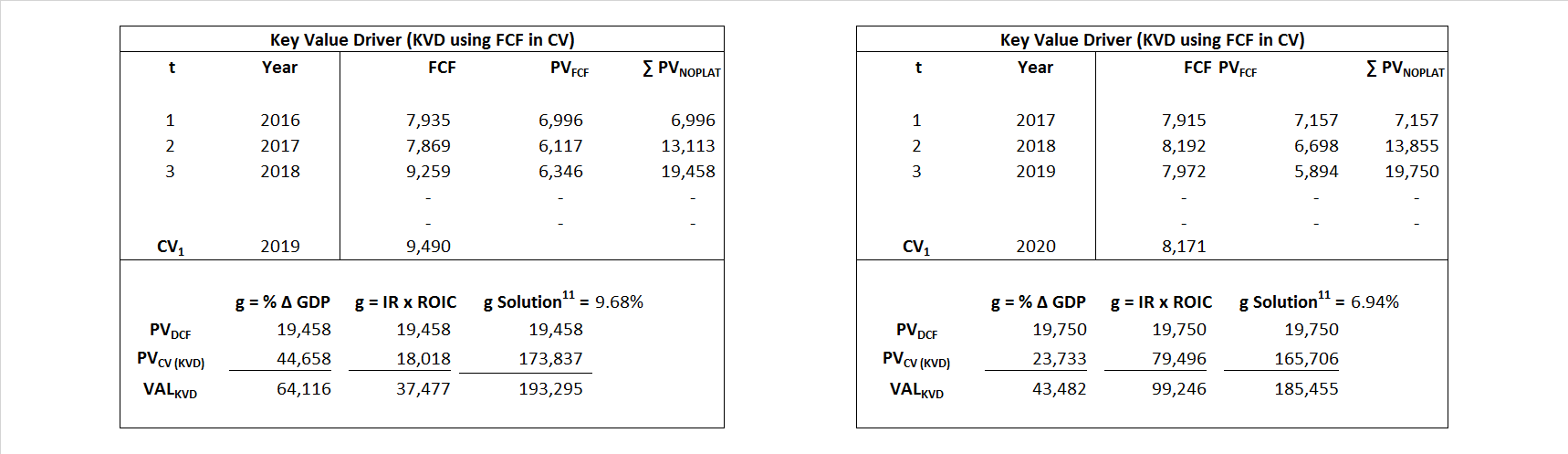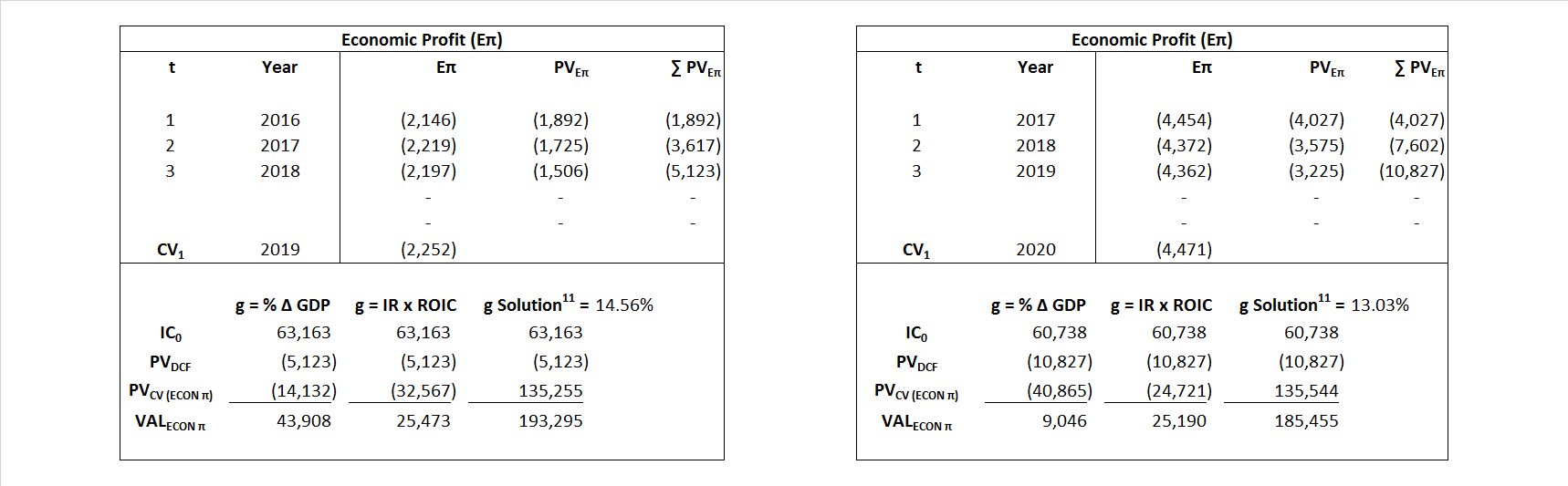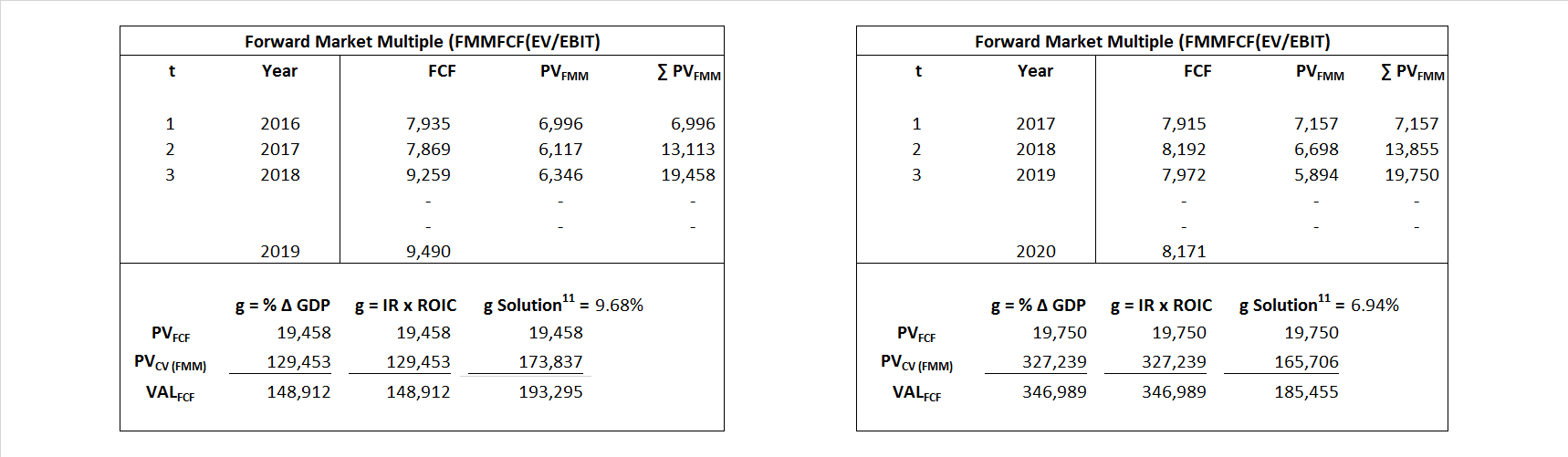# Coca-Cola

## Analyst Listing

The following analysts provide coverage for the subject firm as of May 2016:

 Broker Analyst Analyst Email William Blair Bhavan Suri bsuri@williamblair.com Stifel Nicolaus Brad R. Reback rebackb@stifel.com Pacific Crest Securities-KBCM Brent Bracelin bbracelin@pacific-crest.com Drexel Hamilton Brian J. White bwhite@drexelhamilton.com JMP Securities Greg Mcdowell gmcdowell@jmpsecurities.com Cowen & Company Gregg Moskowitz gregg.moskowitz@cowen.com DA Davidson Jack Andrews jandrews@dadco.com Jefferies John DiFucci jdifucci@jefferies.com SunTrust Robinson Humphrey John Rizzuto john.rizzuto@suntrust.com Stephens Inc Jonathan B. Ruykhaver jonathan.ruykhaver@stephens.com Atlantic Equities Josep Bori j.bori@atlantic-equities.com Deutsche Bank Research Karl Keirstead karl.keirstead@db.com BMO Capital Markets Keith Bachman keith.bachman@bmo.com Evercore ISI Kirk Materne kirk.materne@evercoreisi.com RBC Capital Markets Matthew Hedberg matthew.hedberg@rbccm.com Raymond James Michael Turits michael.turits@raymondjames.com Maxim Group Nehal Chokshi nehalchokshi@techinsightsresearch.com Credit Suisse Philip Winslow philip.winslow@credit-suisse.com Canaccord Genuity Richard Davis Jr. rdavis@canaccordgenuity.com Guggenheim Securities Ryan Hutchinson ryan.hutchinson@guggenheimpartners.com Needham Scott Zeller szeller@needhamco.com Oppenheimer Shaul Eyal shaul.eyal@opco.com FBN Securities Shebly Seyrafi sseyrafi@fbnsecurities.com Mitsubishi UFJ Securities (USA) Stephen D. Bersey sbersey@us.sc.mufg.jp Wedbush Securities Steve Koenig steve.koenig@wedbush.com Northland Securities Tim Klasell tklasell@northlandcapitalmarkets.com Bernstein Research Ali Dibadj ali.dibadj@bernstein.com BMO Capital Markets Amit Sharma amit.sharma@bmo.com Deutsche Bank Research Bill Schmitz Jr. william.schmitz@db.com Wells Fargo Securities Bonnie Herzog bonnie.herzog@wellsfargo.com Consumer Edge Research Brett Cooper bcooper@consumeredgeresearch.com Jefferies Kevin Grundy kgrundy@jefferies.com Stifel Nicolaus Mark D. Swartzberg mswartzberg@stifel.com RBC Capital Markets Nik Modi nik.modi@rbccm.com Susquehanna Financial Group Pablo Zuanic pablo.zuanic@sig.com Evercore ISI Robert Ottenstein robert.ottenstein@evercoreisi.com Cowen & Company Vivien Azer vivien.azer@cowen.com SunTrust Robinson Humphrey William B. Chappell Jr. bill.chappell@suntrust.com

## Primary Input Data## Derived Input Data

### Equational Form

Net Operating Profit Less Adjusted Taxes NOPLAT  6,313   2,226$NOPLAT\, =\, EBIT\, x\, (1 \,-\, Avg \,\,Tax\,\, Rate\,\, on\,\, EBIT)$
Free Cash Flow FCF  7,975  6,534$FCF\,=NOPLAT\,+\,Non-Cash\,Expenses-\Delta NWC\,-\,NCS$
Tax Shield TS  196  154$TS\,=\,Interest\,\,Paid\,\,x\,\, Avg \,\,Tax\,\,Rate\,\, on\,\, Pre-Tax\,\, Income$
Invested Capital IC  63,163  60,738$IC\,=\,Fixed\,\,Operating\,\,Assets\,\,+\,\,Net\,\, Working\,\, Capital$
Return on Invested Capital ROIC 9.99% 3.66%$ROIC\,=\,\frac { NOPLAT }{ IC }$
Net Investment NetInv  5,484  (1,690)$NetInv\,=\,{ {IC}_{1}}-{{IC}_{0}}+Depreciation$
Investment Rate IR 86.87% -75.93%$IR\,=\,\frac {NetInv}{NOPLAT}$
Weighted Average Cost of Capital WACCMarket 13.42% 10.59%$WACC\,=\,\frac { E }{ V } { R }_{ E }\,+\,\frac { P }{ V } { R }_{ P }\,+\,\frac { D }{ V } { R }_{ D }\left( 1- Avg\,\, Tax\,\,Rate\,\,on\,\,Pre-Tax\,\,Income \right)$
WACCBook  6.60% 6.31%
Enterprise value EVMarket  193,295  185,455$EV\,=\,Market\,\,Cap\,\,Equity\,+\,\,Long\,\,Term\,\,Debt\,-\,Cash$
EVBook  191,965  185,263
EV/EBIT Multiple$\frac{EV_{Market}}{EBIT}$  19.90  54.16$EV/EBIT\,=\,\frac { EV}{ EBIT}$
Long-Run Growth g = IR x ROIC
8.68%   -2.78% Long-run growth rates of the income variable  are used in the Continuing Value portion of the valuation models.
g = %$\Delta$ GDP   2.50%   2.50%

## Valuation Model Outcomes

The outcomes presented in this study are the result of original input data, derived data, and synthesized inputs and, depending on the equational form of any particular valuation model, may result in irrelevant or implausible results.  For example, in the event WACC < g, the value of this term, often found in the denominator of an equation’s continuation value term, will be expressly negative and may result in a negative overall valuation for the firm.  In the event of a WACC < g relation, the model form as applied to the subject firm offers an irrelevant outcome.

### Equational form

Key Value Driver (NOPLAT) KVD (NOPLAT)${ Value }_{ DCF/KVD }=\sum { \frac { NOPLAT_{ t } }{ { \left( 1+WACC \right) }^{ t } } +\frac { \frac { { NOPLAT }_{ 1 }\left( 1-\frac { g }{ ROIC } \right) }{ WACC-g } }{ { \left( 1+WACC \right) }^{ t } } }$Key Value Driver (FCF) KVD (FCF)${ Value }_{ DCF/KVD }=\sum { \frac { FCF_{ t } }{ { \left( 1+WACC \right) }^{ t } } +\frac { \frac { { NOPLAT }_{ 1 }\left( 1-\frac { g }{ ROIC } \right) }{ WACC-g } }{ { \left( 1+WACC \right) }^{ t } } }$Free Cash Flow FCF${ Value }_{ DCF/FCF }=\sum { \frac { FCF_{ t } }{ { \left( 1+WACC \right) }^{ t } } +\frac { \frac { { FCF }_{ 1 }}{ WACC-g } }{ { \left( 1+WACC \right) }^{ t } } }$Economic Profit ECON π${ Value }_{ { ECON\pi } }= I{ C }_{ 0 }+\sum { \frac { { IC }_{ t-1 }(ROI{ C }_{t}-WAC{C}_{t}) }{ { \left( 1+WACC \right) }^{ t } }+ \frac {\frac { I{C}_{0}\ x\ (ROI{C}_{1}\ -\ WAC{C}_{1}) }{ WACC-g } }{ { \left( 1+WACC \right) }^{ t } } }$Adjusted Present Value APV${ Value }_{ APV }=\sum { \frac { FCF_{ t } }{ { \left( 1+{ k }_{ u } \right) }^{ t } } +\frac { \frac { { FCF }_{ 1 }}{ { k }_{ u }-g } }{ { \left( 1+{ k }_{ u } \right) }^{ t } } } +\sum { \frac { { TS }_{ t } }{ { \left( 1+{ k }_{ tax } \right) }^{ t } } +\frac { \frac { { TS }_{ 1 }}{ { k }_{ tax }-g } }{ { \left( 1+{ k }_{ tax } \right) }^{ t } } }$Forward Market Multiple FMM${ Value }_{ DCF/FMM}=\sum { \frac { FCF_{ t } }{ { \left( 1+WACC \right) }^{ t } } +\frac { { EBIT }_{ 1 }\,{x}\,{FMM}}{ { \left( 1+WACC \right) }^{ t } } }{\,\,\,; \,\,FMM\,=\,\frac{{EV}_{t=0}}{{EBIT}_{t=0}}}$